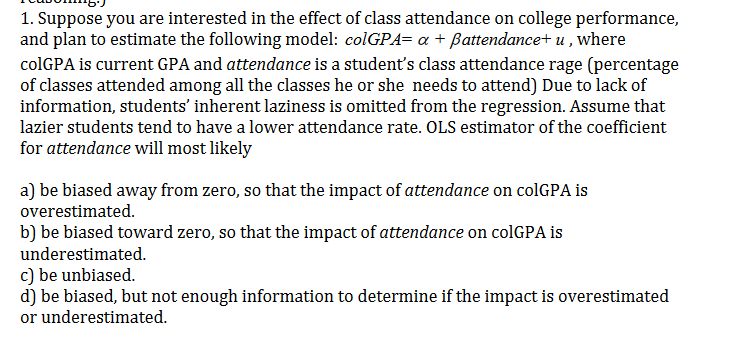# 1. Suppose you are interested in the effect of class attendance on college performance, and plan to estimate the following model: colGPA= a Battendance+ u,where colGPA is current GPA and attendance is a student's class attendance rage (percentage of classes attended among all the classes he or she needs to attend) Due to lack of information, students' inherent laziness is omitted from the regression. Assume that lazier students tend to have a lower attendance rate. OLS estimator of the coefficient for attendance will most likely a) be biased away from zero, so that the impact of attendance on colGPA is overestimated b) be biased toward zero, so that the impact of attendance on colGPA is underestimated c) be unbiased. d) be biased, but not enough information to determine if the impact is overestimated or underestimated

Questionhelp_outlineImage Transcriptionclose1. Suppose you are interested in the effect of class attendance on college performance, and plan to estimate the following model: colGPA= a Battendance+ u,where colGPA is current GPA and attendance is a student's class attendance rage (percentage of classes attended among all the classes he or she needs to attend) Due to lack of information, students' inherent laziness is omitted from the regression. Assume that lazier students tend to have a lower attendance rate. OLS estimator of the coefficient for attendance will most likely a) be biased away from zero, so that the impact of attendance on colGPA is overestimated b) be biased toward zero, so that the impact of attendance on colGPA is underestimated c) be unbiased. d) be biased, but not enough information to determine if the impact is overestimated or underestimated fullscreen